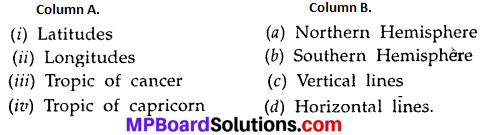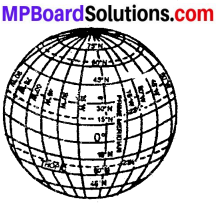# MP Board Class 6th Social Science Solutions Chapter 7 Latitudes and Longitudes

## MP Board Class 6th Social Science Solutions Chapter 7 Latitudes and Longitudes

### MP Board Class 6th Social Science Solutions Chapter 7 Text Book Exercise

MP Board Class 6th Social Science Solutions Chapter 7 Short Answer Type Questions

Question 1.
Question (a)
Why latitudes and longitudes have been drawn on a globe map?
To locate the exact position of places on earth, latitudes and longitudes lines are drawn on a globe or a map. We can easily locate the geographical position of any village, city, town, country, or any place with the help of these lines.

Question (b)
What are latitudes? Name the main latitude?
1. The spherical and horizontal lines drawn parallel to the equator are called latitudes.

2. There are 90 latitudes in the northern hemisphere and 90 latitudes in the southern hemishpere. In all there are 180 latitudes drawn on the globe. The equator is the largest latitude drawn on the globe. The equator is also known as the 0° latitude.

3. The 23$$\frac{1}{2}$$° latitude north, in the northern hemisphere is called the tropic of cancer.

4. Similarly the 23$$\frac{1}{2}$$° latitude south, in the southern hemisphere is called the tropic of capricorn.

5. The Arctic Circle lies at the distances of 66$$\frac{1}{2}$$° N of the equator.

6. The Antarctic Circle 66$$\frac{1}{2}$$° S is similar to the Arctic Circle but lies in the southern hemisphere.Question (c)
What are longitudes? Name the main longitude of the main meridian?

1. The vertical lines drawn from north to south are called longitudes.
2. The longitude passes through Greenwich Observatory near London in England is called 0° longitude, Prime Meridian.
3. The other longitudes are drawn east and west of the Prime Meridian.
4. The Prime Meridian divides the world into Eastern (E) and Western (W) hemisphere.

Question (d)
What are the differences between latitudes and longitudes?
Latitudes:

1. The line drawn horizontally parallal to the equator on the globe is latitude.
2. All the horizontally parallel lines are not in equal size.
3. There are 180 latitude lines.

Longitudes:

1. The verticle lines on the globe are longitudes.
2. All the verticle lines are equal in size.
3. There are 360 longitudes lines.

Question (e)
Why the tropic of cancer and the tropic of capricorn has been drawn at 23$$\frac{1}{2}$$°?
1. The earth is tilted 23$$\frac{1}{2}$$°on its axis. Therefore, the sun shines straight only once in a year on 23$$\frac{1}{2}$$° northern and southern hemispheres.

2. The sun never shines directly from this latitudes to the northern or southern hemispheres. Therefore, the tropic of cancer and tropic of capricorn has been determined at 23$$\frac{1}{2}$$°.

MP Board Class 6th Social Science Solutions Chapter 7 Long Answer Type Questions

Question 2.
Question (a)
What are latitudes and longitudes. Write the characteristics of these lines?
1. Latitudes:
The spherical and horizontal lines drawn parallel to the equator are called latitudes.

Characteristics of the Latitudes:

1. These lines are drawn parallel to the equator from east to west.
2. They are spherical.
3. The distance between two latitudes is the same.
4. The size of the latitudes decrease as we go towards the poles. The poles become just points.
5. The length of the latitudes are not same.
6. The area from north to the equator is called northern hemisphere and that from south to the equator is called southern hemisphere.

2. Longitudes:
The vertical lines drawn from north to south are called longtitudes.

Characteristics of Longitudes:

1. The longtitudes are semi – circular.
2. Their length are the same.
3. The distance between the longitudes is the maximum at the equator, and the distance decreases as we move towards the poles.
4. The longitudes are drawn at 1° interval from the prime meridian. They are 360 in number.Question (b)
What are equator and prime meridian? In how many hemispheres the world has been divided by these lines?
1. Equator:
Equator is an imaginary line which is drawn on the globes (the model of earth) in such a way that at all points it lies exactly half way between the north pole and the south pole.

2. Prime Meridian:

• Like the main latitude (0° equator), there is a main longitude. This longitude passes through Greenwich Observatory near Landon in England. This is called 0° longitude, Prime Meridian.
• The world has been divided by these lines into four hemisphere.Question 3.

Fill in the blanks:

1. All latitudes are parallel to the ……………….
2. The uppermost point on the north of the world is called ……………. pole and lower most on the south of the world is called ……………….. pole.
3. The main meridian is also called the ……………..
4. The equator divides the world into ……………. and ………………. hemisphere.
5. The total number of longitudes are ……………..

1. equator
2. north, south
3. Prime Meridian
4. northern, southern
5. 360.Question 4.
Match the column(i) (d) Horizontal lines
(ii) (c) Vertical lines
(iii) (a) Nothern Hemisphere
(iv) (b) Southern Hemisphere

Question 5.
Question (a)
The biggest latitude is called?
(i) tropic of cancer
(ii) equator
(iii) tropic of capricorn
(iv) prime meridian
(ii) equator.

Question (b)
The distance between the longitudes towards the poles?
(i) increase
(ii) decrease
(iii) parallel
(iv) none of these